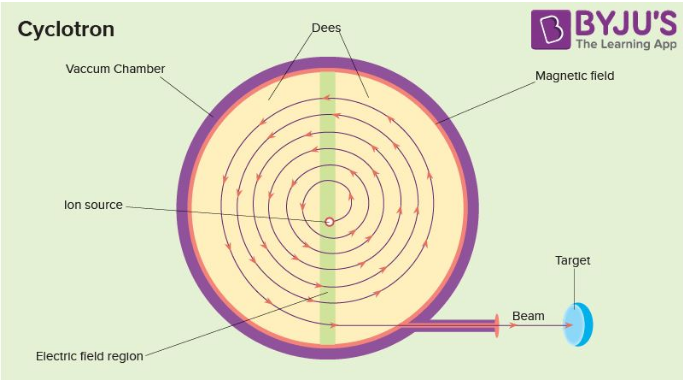# MCQ on Cyclotron for NEET 2021

Cyclotron can be defined as a type of particle accelerator in which charged particles accelerate outwards from the centre along a spiral path. These particles are held to a spiral trajectory by a static magnetic field and accelerated by a rapidly varying electric field.The Principle of Cyclotron is that when a particle is moving normal to the magnetic field it will experience a Lorentz force because of which the particle moves in a circular path. This is explained in detail below

1. The dees are placed opposite each other with a narrow gap between them. The particles can move in the gap. The particles are put in the centre of the gap.
2. The dees are kept between the two poles of an electromagnet.
3. The magnetic field causes the particles to take a circular path due to the Lorentz force
4. A very high alternating voltage is applied between the dees. The voltage creates an alternating field which accelerates the particles.
5. The frequency of the voltage is adjusted such that the particles make one circle during one cycle of voltage. The frequency must be set to the particle’s cyclotron frequency.

The expression for Frequency, f=qB/2πm

Particle Energy, E=q2B2R2/2m

where,

B is the magnetic field strength

Q is the electric charge of the particle

m is the relativistic mass of the charged particle

R is the maximum radius of the circular path followed by the positive ion

Q1: The angular frequency of a cyclotron is independent of

1. Speed
2. Mass
3. Magnetic field
4. Charge

Q2: The maximum kinetic energy of the positive ion in the cyclotron is

1. qBR2/2m
2. q2B2R2/2m
3. q2B2R2/m
4. qBR/m

Q3: Cyclotron cannot accelerate

1. Electrons
2. Neutrons
3. Positive ions
4. (Both (1) and (2)

Answer: (d) Both (1) and (2)

Q4: The cyclotron frequency of an electron grating in a magnetic field of 1 T is approximately

1. 28 MHz
2. 280 MHz
3. 2.8 GHz
4. 28 GHz

Q5: Suppose a cyclotron is operated at an oscillator frequency of 12 MHz and a dee radius of 53cm. What is the resulting kinetic energy of the deuterons?

1. 16.6 MeV
2. 12 MeV
3. 15 MeV
4. 14 MeV

Q6: An alternating electric field of frequency f is applied across the dees (radius = R) of a cyclotron that is being used to accelerate protons (mass = m). The operating magnetic field (B) used in the cyclotron and the kinetic energy (K) of the proton, produced by it, are given by

1. B = mf/e and K= 2mπ2v2R2
2. B =2πmf/e and K= m2πvR2
3. B =2πmf/e and K= 2mπ2v2R
4. B = mf/e and K= m2πvR2

Answer: (c) B =2πmf/e and K= 2mπ2v2R

Q7: The energy of emergent protons in MeV from a cyclotron having a radius of its dees 2 m and applied magnetic field 0.8 T is (mass of proton = 1.67 x 10-27 kg).

1. 0.961 x 10-11 J
2. 1.22 x 10-11 J
3. 1.5 x 10-12 J
4. 12 x 10-11 J

Answer: (a) 0.961 x 10-11 J

Q8: Cyclotron can be used in

1. Particle therapy to treat cancer
2. Source of high energy beam for a nuclear physics experiment
3. Produce short-lived positron-emitting isotopes for PET imaging
4. All the above

Q9: A proton of energy 100 eV is moving perpendicular to a magnetic field 10-4 T. The cyclotron frequency of the proton in radian/sec

1. 2.80 x 106
2. 9.6 x 103
3. 5.6 x 106
4. 1.76 x 106

Q10: A cyclotron can accelerate

1. β particles
2. α particles
3. High-velocity gamma rays
4. High-velocity X-rays